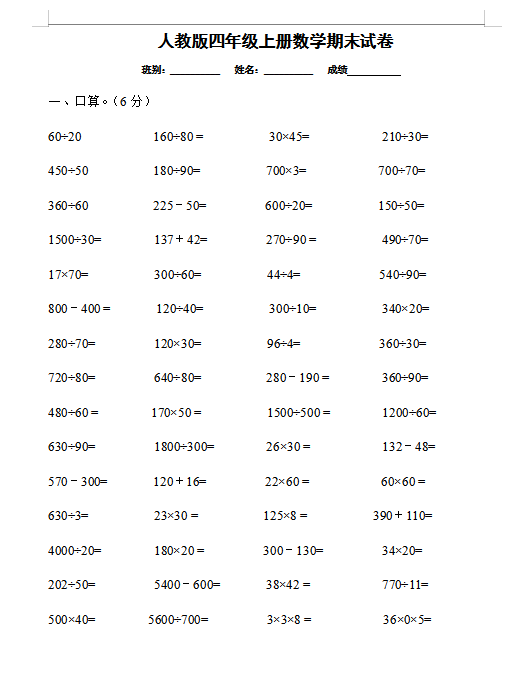60÷20           160÷80 =          30×45=           210÷30=

450÷50          180÷90=          700×3=           700÷70=

360÷60          225－50=         600÷20=          150÷50=

1500÷30=        137＋42=         270÷90 =          490÷70=